# dedecms二次开发：织梦SQL数据库类详解

2015-04-17 14:19 阅读 83 次 评论关闭

## 1.创建数据表

这里我们这个数据库叫dedebbs,表前缀用的默认的"dede_",我们用Navicat for MySQL来创建一个名为"dede_test"的表,里面就2个字段,1个id,作为唯一标识,另一个name作为名称(图1).然后往里面添加测试数据(图2),这个操作类似于Access或者EXCEL,比较简单,这里不做冗述.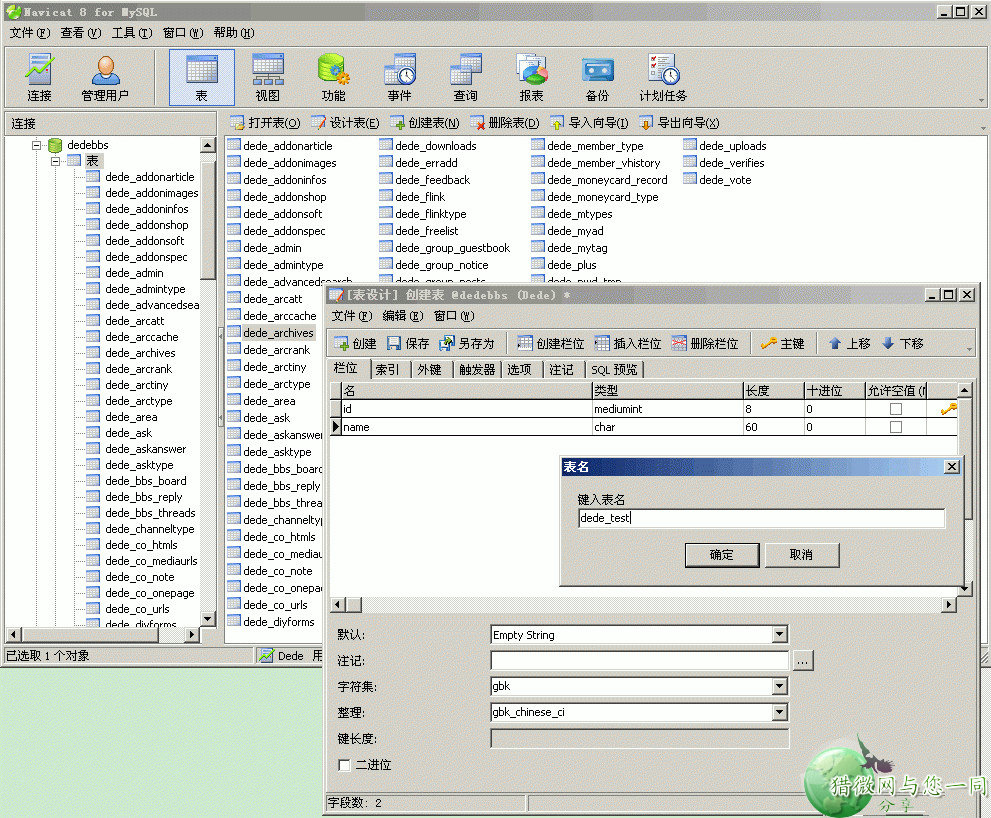(图1)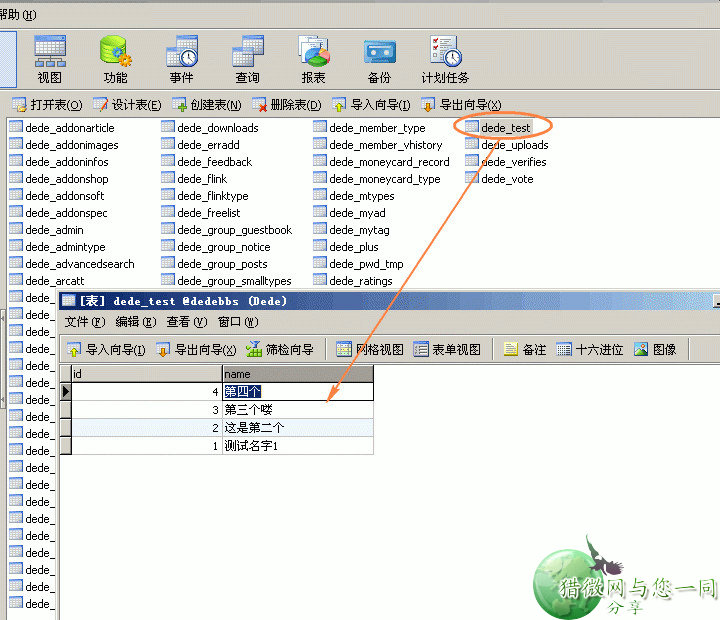(图2)

这里需要注意的是,我们采用的是gbk版本的程序,所以数据表相关的编码也要采用相应版本的编码,在表的"选项"中设置为gbk相关.下面我将sql列出,如果懒得去创建表,可以在dedecms系统后台的命令运行器中执行下: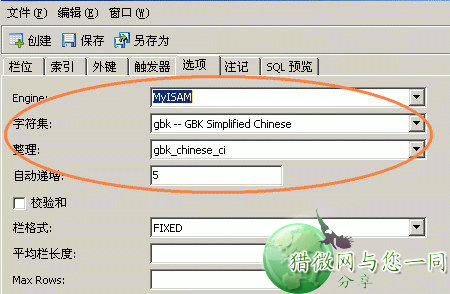(图3)

-- ----------------------------
-- 表 `dede_test`结构
-- ----------------------------
DROP TABLE IF EXISTS `dede_test`;
CREATE TABLE `dede_test` (
`id` mediumint(8) NOT NULL AUTO_INCREMENT,
`name` char(60) NOT NULL DEFAULT '',
PRIMARY KEY (`id`)
) ENGINE=MyISAM AUTO_INCREMENT=5 DEFAULT CHARSET=gbk;
-- ----------------------------
-- 插入到 dede_test中的数据
-- ----------------------------
INSERT INTO `dede_test` VALUES ('1', '测试名字1');
INSERT INTO `dede_test` VALUES ('2', '这是第二个');
INSERT INTO `dede_test` VALUES ('3', '第三个喽');
INSERT INTO `dede_test` VALUES ('4', '第四个');

## 2.连接数据库,查询数据表

表"dede_test"我们已经创建完成了,接下来我们要连接数据库了,其实方法非常简单.我们在网站根目录中创建一个test.php的文件,这个文件最好也用gbk的编码,可以用专门的编辑器来创建文件.

test.php
-------------------------------
<?php
require_once (dirname(__FILE__) . "/include/common.inc.php");
print_r(\$dsql);
?>
--------------------------------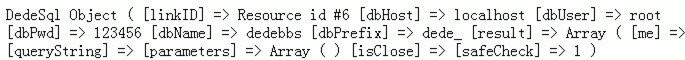(图4)

DedeSql Object
(
[linkID] => Resource id #6
[dbHost] => localhost
[dbUser] => root
[dbPwd] => 123456
[dbName] => dedebbs
[dbPrefix] => dede_
[result] => Array
(
[me] => 0
)

[queryString] =>
[parameters] => Array
(
)

[isClose] =>
[safeCheck] => 1
)

test.php
-------------------------------
<?php
require_once (dirname(__FILE__) . "/include/common.inc.php");
if(\$dsql->IsTable('dede_test')){
//如果存在dede_test表 by www.daxuewen8.com
//-------------------
//|    查询一条记录 |
//|    GetOne()     |
//-------------------
//        ↓
\$row = \$dsql->GetOne("SELECT * FROM dede_test WHERE id = 3");
print_r(\$row);
}
?>
--------------------------------

Array
(
[id] => 3
[name] => 第三个喽
)

Tip:其实我们可以深入的研究下dedesql.class.php这个类,258行就有这个方法:

//执行一个SQL语句,返回前一条记录或仅返回一条记录
function GetOne(\$sql='',\$acctype=MYSQL_ASSOC)
{
global \$dsql;
if(\$dsql->isClose)
{
\$this->Open(false);
\$dsql->isClose = false;
}
if(!empty(\$sql))
{
if(!eregi("limit",\$sql)) \$this->SetQuery(eregi_replace("[,;]\$",'',trim(\$sql))." limit 0,1;");//这里用正则看看limit是否存在,如果不存在的话就自动加上limit 0,1这个内容,关于limit的介绍可以去看看SQL关于这个的介绍
else \$this->SetQuery(\$sql);
}
\$this->Execute("one");
\$arr = \$this->GetArray("one",\$acctype);
if(!is_array(\$arr))
{
return '';
}
else
{
@mysql_free_result(\$this->result["one"]); return(\$arr);//清空资源,返回这个数组
}
}

## 3.深入查询,继续研究SELECT

test.php
-------------------------------
<?php
require_once (dirname(__FILE__) . "/include/common.inc.php");
if(\$dsql->IsTable('dede_test')){
//如果存在dede_test表
//-------------------
//|    查询一条记录 |
//|    GetOne()     |
//-------------------
//        ↓
\$row = \$dsql->GetOne("SELECT * FROM dede_test WHERE id = 3");
echo "查询id=3的记录:<br />显示结果:";
print_r(\$row);
//-------------------
//|    查询多条记录 |
//|    Execute()     |
//-------------------
//        ↓
echo "<hr />查询dede_test表中的所有记录:<br />显示结果:<br />";
\$sql = "SELECT * FROM dede_test";
\$dsql->Execute('me',\$sql);
while(\$arr = \$dsql->GetArray('me'))
{
echo "id = {\$arr['id']} ,name = {\$arr['name']}<br />";
}
}
?>
--------------------------------

id = 1 ,name = 测试名字1
id = 2 ,name = 这是第二个
id = 3 ,name = 第三个喽
id = 4 ,name = 第四个

while(\$dbobj = \$dsql->GetObject('me'))
{
echo "id = {\$dbobj->id} ,name = {\$dbobj->name}<br />";
}

 tips:这里查询完了其实还可以用\$dsql->GetTotalRow("me")来获取下查询出来的总数.

## 4.插入删除,也要用到SQL

<hr />

<form action="test.php" type="post">
<input type="hidden" name="dopost" value="save">

<input name="提交" type="submit" value="提交">
</form>

//-------------------
//|    表单处理过程 |
//|    Save()     |
//-------------------
//        ↓
empty(\$dopost)? "" : \$dopost;
if(\$dopost == "save"){
//如果执行插入操作
\$sql = "INSERT INTO `dede_test` (`name`) VALUES ('{\$name}')";
\$dsql->ExecuteNoneQuery(\$sql); //执行这个插入语句
\$lastInsertID = \$dsql->GetLastID(); //获取插入后的最后的ID,然后再传给下一个页面
ShowMsg("成功增加一条记录内容！","test.php?id={\$lastInsertID}");
exit();
}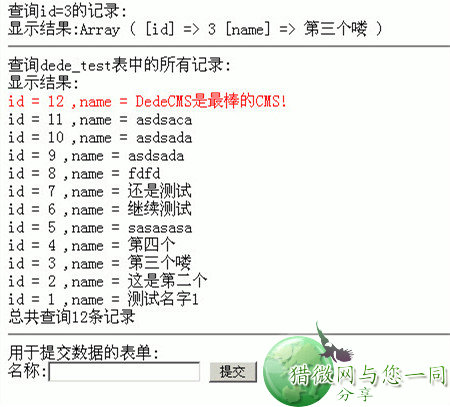(图5)

while(\$dbobj = \$dsql->GetObject('me'))
{
if(\$id==\$dbobj->id){
//如果传递的id值和查询值相同,则高亮显示最后一条插入记录
echo "<font color='red'>id = {\$dbobj->id} ,name = {\$dbobj->name}</font> <a href='test.php?dopost=del&id={\$dbobj->id}'>[删除]</a>    <br />";//这里增加了一个删除的超链接
}else{
echo "id = {\$dbobj->id} ,name = {\$dbobj->name} <a href='test.php?dopost=del&id={\$dbobj->id}'>[删除]</a><br />";
}
}

empty(\$dopost)? "" : \$dopost;
if(\$dopost == "save"){
//如果执行插入操作
\$sql = "INSERT INTO `dede_test` (`name`) VALUES ('{\$name}')";
\$dsql->ExecuteNoneQuery(\$sql);
\$lastInsertID = \$dsql->GetLastID();
ShowMsg("成功增加一条记录内容！","test.php?id={\$lastInsertID}");
exit();
}elseif(\$dopost == "del"){
//如果dopost为del,则删除数据
\$id = isset(\$id) && is_numeric(\$id) ? \$id : 0;
\$sql = "DELETE FROM `dede_test` WHERE (`id`='{\$id}')";
\$dsql->ExecuteNoneQuery(\$sql);
ShowMsg("成功删除一条记录内容！","test.php");
exit();
}

 tips:这里用到了一个ShowMsg()函数,这个是用来返回一个对话框的,详细可以查看下\include\common.func.php的372行相关函数的代码.

这里我们结合了DedeCMS程序的dedesql类讲解了这个类的常见的用法,我们不难发现用这个类操作数据库是非常方便的,当然这个需要用户对sql比较熟悉.今天我们就讲解到这里了,下次有机会再结合datalist类和模板引擎来介绍织梦二次开发相关的内容.